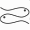# Bifolium

The bifolium is a classical plane curve given by

f(x,y) = (x^2 + y^2 )^2 -a*x^2*y.

In polar coordinates, this curve is given parametrically as

r(theta) = a sin(theta) [cos(theta)]^2.

The parameter a scales the level set, but does not change its topology.

Previous: ModelsThe Pisces Home Page